# Electronics and Communication Engineering - Exam Questions Papers

36.

Consider the program segment written for 8085 based system:
LXI S, FFFFH
MVI A, 00H
MVI B, 0FH
SUB B
CNC GOLOC
MVI A, FFH
where in the program subroutine labelled ''GOLOC'' introduces delay of 28 T-states only. How many T-states are required to execute above program statement?

 A. 41 B. 69 C. 44 D. Indeterminate

Explanation:

Since carry (CY) flag will not be set at the execution of "SUB B" instruction;

check for CY flag fails but this requires 9(= 6 OP - CODE FETCH + 3T - states) T-states

Total T-states = 10 + 7 + 7 + 4 + 9 + 7 = 44.

37.

A discrete random variable X takes values from 1 to 5 with probabilities as shown in the table. A student calculates the mean of X and 3.5 and her teacher calculates the variance of X as 1.5. Which of the following statements is true?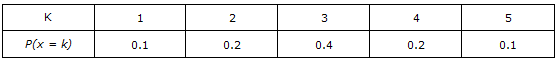A. Both the student and the teacher are right B. Both the student and the teacher are wrong C. Both the student is wrong but the teacher is right D. The student is right but the teacher is wrong

Explanation:

The mean is 3.

38.

The effective modulation index is:

 A. 0.91287 B. 0.8306 C. 1 D. none of these

Explanation: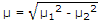μ1 = 0.2 x 3 = 0.6

μ2 = 0.2 x 4 = 0.8

μ = (0.36) + (0.64) = 1.

39.

Consider Continuous time system y(t) =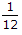x(t) -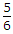. For the system to be linear response ax1(t) + bx2(t) should be ay1(t) + by2(t) with relation between constant 'a' and 'b' as __________ .

 A. a + b = 2 B. a x b = 1 C. a = b D. a + b = 1

Explanation:

Let ax1(t) + bx2(t) = m(t)

where m(t) is new input which causes the output n(t)

n(t) =m(t) -n(t) ={ax1(t) + bx2(t)} -add and subtract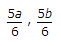n(t) =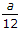x1(t) -a +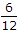x2(t) -b +a +b -n(t) =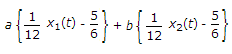+{a + b - 1}

n(t) = ay1(t) + by2(t) +{a + b - 1}

For linearity, n(t) = ay1(t) + by2(t){a + b - 1} = 0 a + b = 1.

40.

Hari (H), Gita (G), Irfan (I) and Saira (S) are sibiligs (i.e. brothers and sisters). All were born on 1st January. The age difference between any two successive siblings (that is born one after another) is less than 3 years. Given the following facts:
(i) Hari's age + Gita's age > Irfan's age + Saira's age.
(ii) The age difference between Gita and Saira is 1 year. However, Gita is not the oldest and Saira is not the youngest.
(iii) There are not twins.
In what order were they born (Oldest first)?

 A. HSIG B. SGHI C. IGSH D. IHSG

Explanation:

(i) H + G > I + S

(ii) |G - S| = 1

Meaning G and S will be next to each other in the order. So the option A is ruled out.

G not oldest

S not youngest

(iii) No twins

Going by the options, we will try solve the equation,

Taking as example with youngest aged 1, we can try to solve the equation, and correct the age (started with ages 4, 3, 2, 1) to suit conditions (i) and (ii) which gives 5, 4, 3, 1

S I + 4 Generalizing, we can take their ages in terms of I's age,

G I + 3 In this case, H + G > I + S

H I + 2 Since 2I + 5 > 21 + 4

I I

I In this order, G is always less than I and H always less than S.

So G > I and H < S

S Implies G + H < I + S, all values are positive

H Defies condition i) Hence incorrect.

I In this order H < I, G < S

H Hence H + G < I + S

S Defies condition (i)

G Hence incorrect.

#### Current Affairs 2022

Interview Questions and Answers